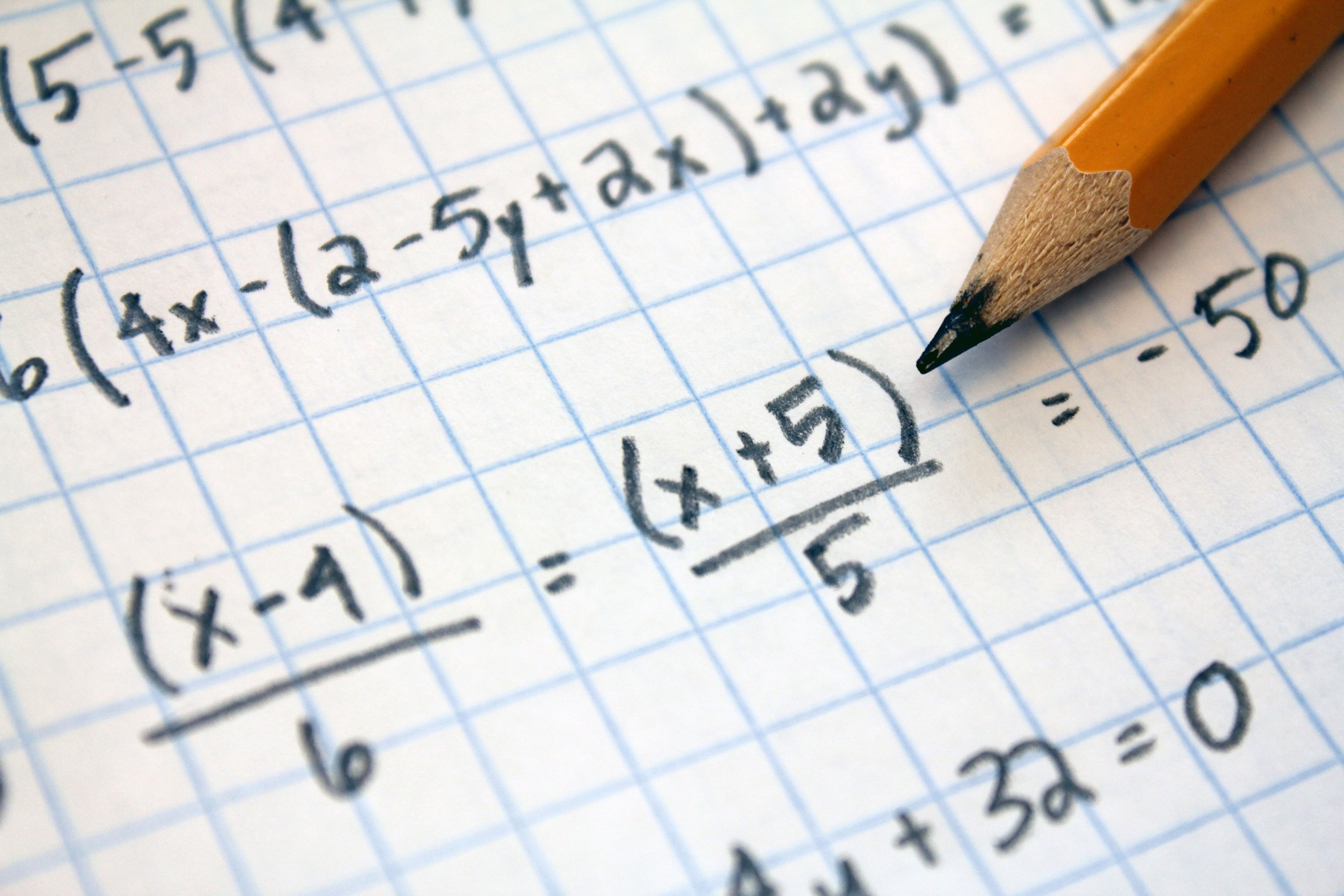# Finding The Factor In Algebra

For basic algebra students in Costa Mesa, they may need a tutor to help them practice and comprehend factoring. There are many different ways to learn more about basic algebra factoring in basic algebra, and with practice, it becomes second nature.## Basic algebra factoring is one of the major lessons learned in basic algebra..

For basic algebra students in Costa Mesa, they may need a tutor to help them practice and comprehend factoring.  There are many different ways to learn more about basic algebra factoring in basic algebra, and with practice, it becomes second nature.

Basic algebra factoring begins in pre-algebra.  Costa Mesa basic algebra students may start with the basics of,

“What is an algebra factor?”

• An algebra factor is a quantity multiplied with others.  An algebra factor is a number or variable, out of at least two numbers or variables, that can be multiplied together to get a particular number or quantity.

## What does that mean for basic algebra students?

Let’s go through a couple of basic algebra examples of factoring numbers, then simple algebra variable expressions.  Most of these examples are lessons taught in pre-algebra in Costa Mesa, so most basic algebra students should be familiar with them.

• What are the algebra factors of 6?
• 2 x 3 = 6, so two and three are both factors of six.
• 1 x 6 = 6, so one and six are also factors of six.
• Answer: 1, 2, 3, and 6 are factors of 6

• What are the algebra factors of 60?
• This one is a little tricky.  There are a number of ways to multiply numbers to get to 60:
• 1 x 60
• 2 x 30
• 3 x 20
• 4 x 15
• 5 x 12
• 6 x 10
• 2 x 2 x 15
• 2 x 3 x 10
• 2 x 3 x 2 x 5
• The list continues, but notice that once we started going into three and four number combinations, the actual factors, or numbers, didn’t increase.  Instead, we began factoring the factors!  With 2 x 30, we can then factor 30 into increasingly smaller numbers.
• Algebra answer:  1, 2, 3, 4, 5, 6, 10, 12, 15, 20, 30, and 60 are all factors of 60.

• What are the algebra factors of 5x + 15?  Then, factor 5x + 15
• As basic algebra students, we need to look at two quantities, instead of just one.  A home algebra tutor can point out, what numbers go into both 5x and 15?  This first step is to find the factors of each one:
• 5x: the factors are 1, 5, and x
• 15: the factors are 1, 3, 5, and 15
• Besides 1, the only factor in common to both quantities is 5.  Therefore, to factor, divide each quantity by 5
• 5x/5 = x and 15/5 = 3
• The final, factored answer is 5(x+3) and the factors are 1, 3, 5, 15, and x

Get yourself on the right track by finding and hiring a weekly home algebra tutor at the start of the school year!

A Plus In Home algebra tutors offers tutoring for all subjects, including basic algebra factoring, in Costa Mesa, Tustin, Huntington Beach, and throughout Orange County.  An algebra Tutor in Costa Mesa can help increase your basic algebra comprehension and your test scores.

Earn an “A” in Algebra this year!  Visit www.FireflyTutors.com.